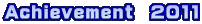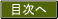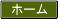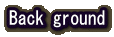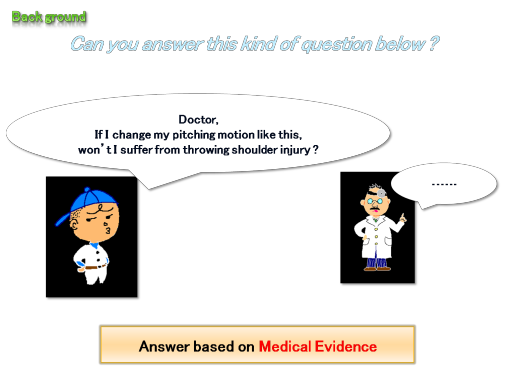Can you answer a kind of question below ?

[Doctor,If I change my pitching motion like this,on’t I suffer from throwing shoulder injury ?]

This is one of the player’s need at a field.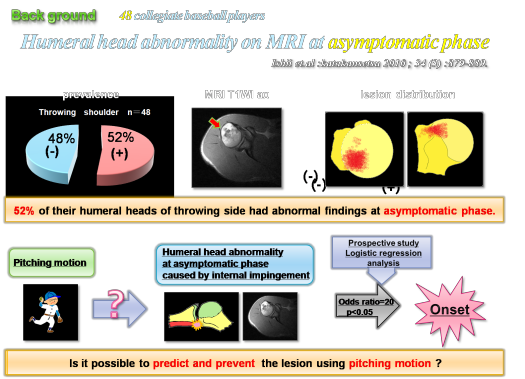We reported the shoulder MRI findings of 48 asymptomatic collegiate baseball players. They underwent conventional MRI at asymptomatic phase.

As a result, 52% of their humeral heads of throwing side had abnormal findings more than the non-throwing side(11%).

The distribution and patterns of these findings were similar to those which have been reported as throwing shoulder injuries in symptomatic shoulders.

Then we investigated who got throwing shoulder injuries after MRI for a year.  We did logistic regression analysis with MRI findings and the data of the onset and found out the factors which significantly affected the onset.

As a result, the odds ratio of the humeral head abnormality was 20. It means that the probability of  the onset of throwing shoulder injuries with humeral head abnormality is 20 times more than without it.

Humeral  head abnormality was thought to be the lesion which was near-related to the onset.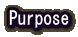The purpose of this study is to predict  the lesion of the humeral head abnormality caused by internal impingement using the pitching motion analysis.

In order to develop the prediction system , we need clarify the following 3 issues.

1. What is the risk-factors on dynamics of humeral head abnormality?

2. Is it possible to predict the lesion existence of humeral head abnormality?

3. Is it possible to predict the lesion distribution of humeral head abnormality?

The subjects were 18 asymptomatic collegiate baseball players who took part in both MRI and pitching motion analysis. The shoulder joint reaction force was calculated by multi-body dynamics analysis with musculoskeletal model. Then we simulated the stress distribution in humeral head at maximum external rotation (MER) of throwing phase using Finite Element Method analysis (FEM). Finally we did logistic regression analysis to identify risk-factors on dynamics which affected humeral head abnormality and we evaluated the validity of the lesion distribution of this simulation using MRI.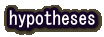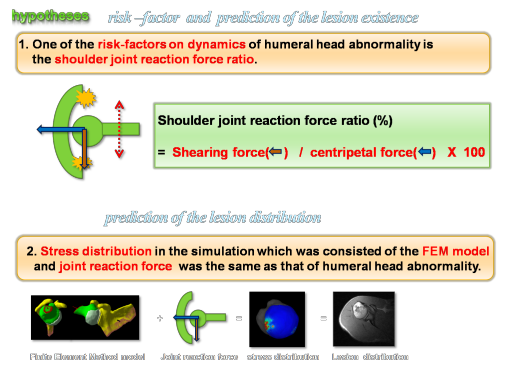We put forward the following 2 hypothesis.

1. One of the risk-factors on dynamics of humeral head abnormality is

the shoulder joint reaction force ratio.

we defined the shoulder joint reaction force ratio as shearing force/centripetal force.

2. Stress distribution in the simulation which was consisted of the FEM model and joint reaction force  was the  same  as  that  of  humeral  head  abnormality .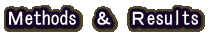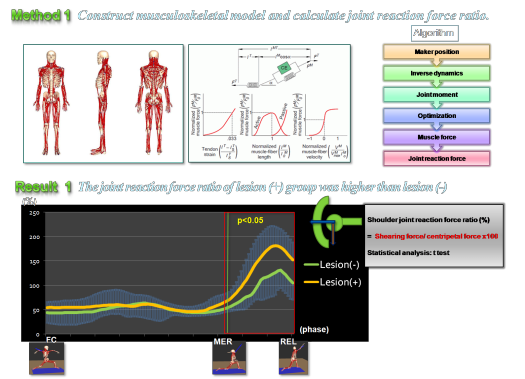Research 1.

method 1:

We constructed the musculoskeletal model . The musculoskeletal model is composed of bones, joints and muscles. It is different from a link-segment model. In

this model, there are almost all muscles around shoulders. Each muscle-tendon actuator has an active contractile element(CE) in parallel with a passive elastic element. “α” shows the pennation angle. These actuators contain some mechanical properties (see above). We can customize these parameters easily and can simulate variously.

We calculated the shoulder joint reaction force ratio by multi-body dynamics analysis with musculoskeletal model and compared the lesion(+) group with the lesion(-) group.

As a result, the shoulder reaction force ratio of the lesion(+) group was significantly higher than that of the lesion(-) group.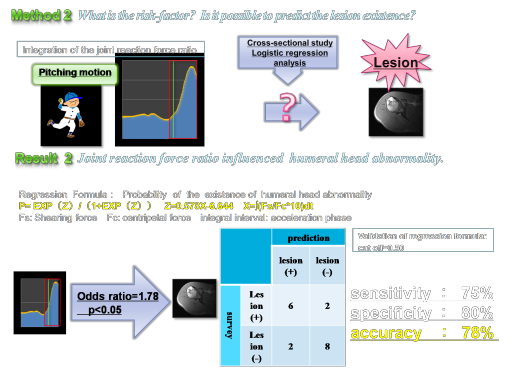Research 2.

What is the risk-factor of the lesion of  humeral  head abnormality. Is it possible to predict the lesion existence?

Method 2:

We did logistic regression analysis with the integration of the joint reaction force during the acceleration phase and MRI findings of humeral head abnormality and found out the factors which significantly affected the lesion. We also calculated regression formula which could predict the probability of the existence of

the lesion and  evaluated the regression model.

As a result, the Odds ratio of  ∫(Fs/Fc*10)dt  was 1.78. It means that the probability of the existence of  humeral head abnormality will increase 1.78 times, if the value of ∫(Fs/Fc*10)dt  increases 10%. We could predict the lesion with 78% accuracy.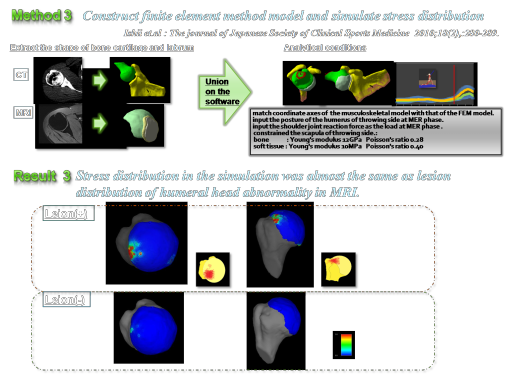Research 3.

We constructed Finite Element Method analysis (FEM) model. In the FEM model , we extracted the shape of bones, cartilages and labrums from CT and MRI. The shoulder joint reaction force was calculated by using multi-body dynamics analysis with musculoskeletal model. We input the force to the FEM model.  We simulated the stress distribution in humeral head at maximum external rotation (MER) of throwing phase using FEM model. Then we evaluated the validity of the simulation

by MRI findings of humeral head abnormality.

As a result, stress  distribution  in  the  simulation  was  almost   the  same  as  that  of  humeral  head  abnormality  in  MRI.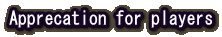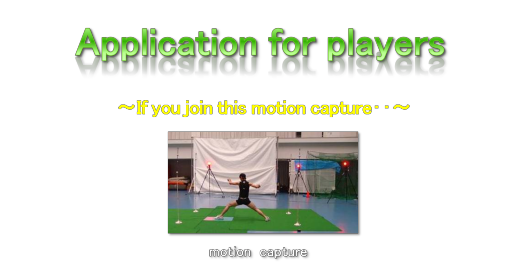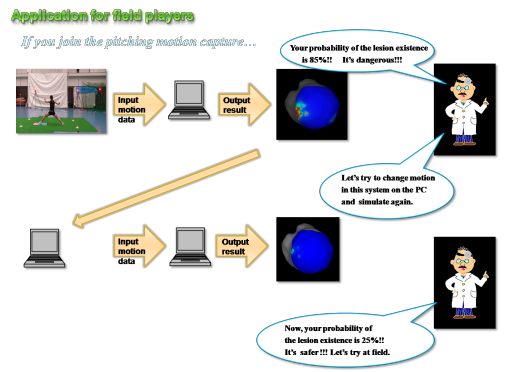If you join the pitching motion capture and use this system, you can know the lesion probability and distribution of the humeral head abnormality of throwing shoulder injury.

Please input motion data (C3D format) to this system, then the lesion distribution & probability of its existence are output.

If there are some problems medically, you can change motion data on a PC just like a game. ex) let’s move elbow down, let’s flex elbow more・・・・・

Please input motion data to this system and simulate again, you can know the lesion probability and distribution of the new motion.

If there is no problem, let’s challenge new pitching motion at field. This system is the Computer Assisted Sports Science.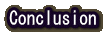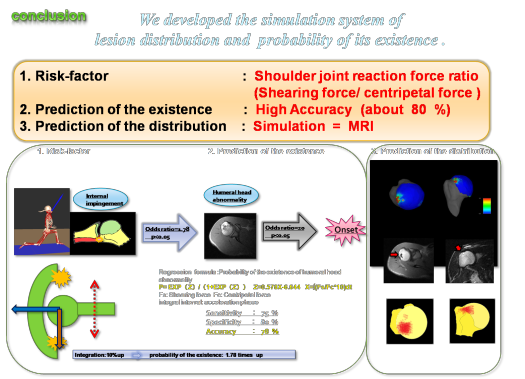We developed the simulation system of the lesion distribution and the probability of the existence of humeral head abnormality caused by internal impingement of throwing shoulder injury.

One of the risk-factors of humeral head abnormality on dynamics was the shoulder joint reaction force ratio (shearing force/centripetal force) at acceleration phase.

We calculated regression formula using the shoulder joint reaction force ratio  and  we could  predict  the  existence of humeral head abnormality with 78% accuracy.

Humeral head abnormality was thought to be the lesion which was near-related to the onset of throwing shoulder injury.

We simulated the stress distribution in humeral head at MER using FEM model input the shoulder reaction force. Stress distribution in the simulation was almost same as that of humeral head

abnormality in MRI. This simulation system was expected to be the useful predictor of the humeral head abnormality.